Next: User's Guide Up: Random Flow Generation Procedure Previous: Method   Contents

# Implementation

Figure 1 shows the main flow-chart of the algorithm. It consists of two functions: (1) spectral generator (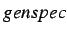) and vector-field generator (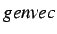). User supplies the input data in form of the size of a spectral sample (integer parameter,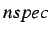), correlation tensor for the vector field and time scale. In case of a turbulent flow modeling this should be the velocity correlation tensor,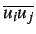and turbulent time scale,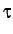. In the case of homogeneous turbulence the correlation tensor and time-scale are constant and are suppled as arguments to thefunction (link A in the figure). In the case of an inhomogeneous turbulence these parameters are supplied to thefunction (link B in the figure). Both cases are implemented in the two different versions of the algorithm (see Sec.3.1). Most of the routines are written in a C language with some auxiliary Fortran routines imported from the Netlib library (www.netlib.org).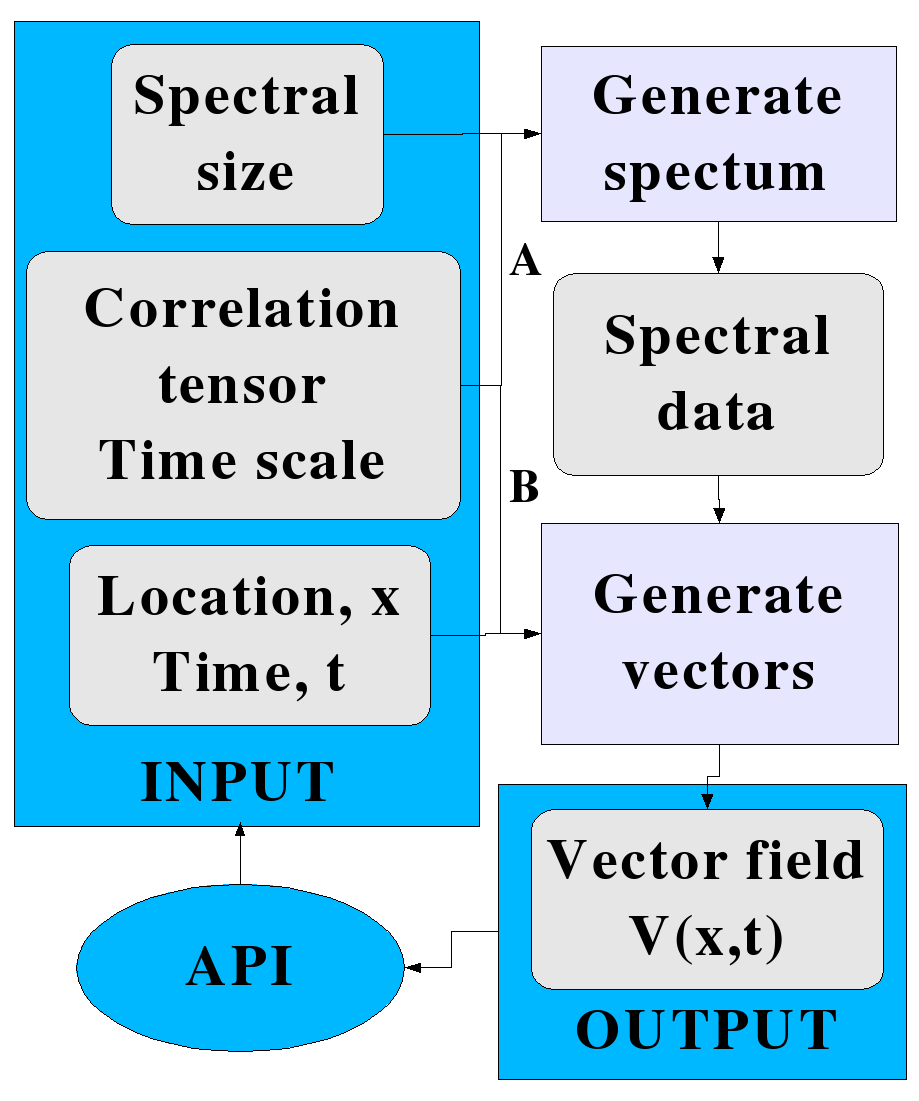User input parameters to the algorithm are shown in Fig.1 and include:- number of spectral modes, 6-dimensional array of velocity correlations,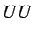(for anisotropic inhomogeneous case: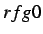), turbulence time scale,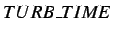, turbulent length scale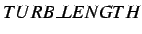(for isotropic homogeneous case), and space-time coordinates,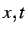. At the beginning there should be only one call tofunction, which initializes the spectral data. These consists of four arrays: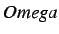,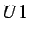,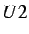, and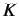. While the first one is the array of scalar values, the other three are all arrays of 3D vectors. These four arrays are global variables and after they are initialized bythey are repeatedly used by thefunction to generate the random vector field. In the homogeneous isotropic version of the algorithm () the user supplies the,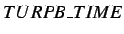, and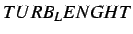parameters tofunction, and then repeatedly calls the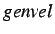functions for different spatial locations and time. In the inhomogeneous anisotropic case (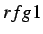), the user callswith only one parameter, defining the size of the spectral sample (), and then repeatedly callswith for different, as well as different values of velocity correlation tensor and time scale. In this way one provides spacial inhomogeneity of the generated vector field. The anisotropy is determined by the correlation tensor.

The complete listing of the main RFG routine is given in the Appendix.Next: User's Guide Up: Random Flow Generation Procedure Previous: Method   Contents
2004-08-27